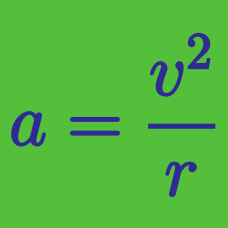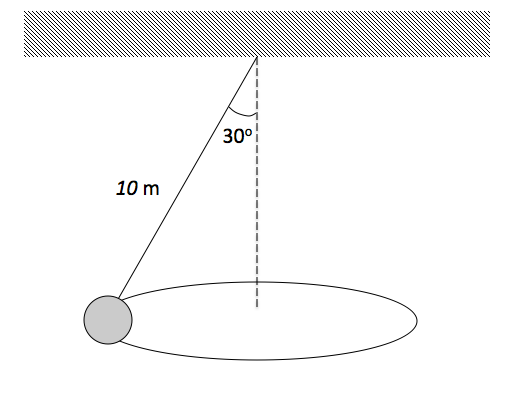Classical Mechanics

# Uniform circular motion - advanced

A pilot executes uniform speed circular path motion in an airplane. The initial velocity (in $\text{m/s}$) of the plane is given by $v_o = 2200 \hat{i} + 2500 \hat{j}.$ One minute later, the velocity of the plane is $v = -2200 \hat{i} - 2500 \hat{j}.$ Find the approximate magnitude of the acceleration of the plane.As shown in the above diagram, a $2 \text{ kg}$ ball swings at a constant speed in a horizontal circle at the end of a cord of length $10 \text{ m}.$ What is the approximate speed of the ball if the rope makes an angle of $30 ^\circ$ with the vertical?

Take gravitational acceleration $g= 10 \text{ m/s}^2.$

A car is in uniform circular motion on a flat circular track of radius $70 \text{ m}.$ If the coefficient of static friction between the car's wheels and the track is $0.5 ,$ approximately how fast can the car move without losing traction with the track?

The gravitational acceleration is $g= 10 \text{ m/s}^2.$

A coin is gently placed on a rotating horizontal turntable that is slowly accelerating. The coin's initial distance from the axis of rotation is $40 \text{ cm}.$ If the coin starts to slip when the coin's speed is $60 \text{ cm/s},$ what is the approximate coefficient of static friction between the coin and the turntable?

The gravitational acceleration is $g= 10 \text{ m/s}^2.$

A particle moves in uniform circular motion on the $xy$-plane. When the particle's position is $(2 \text{ m}, 2 \text{ m} ),$ its velocity is $-4\hat{x} \text{ m/s}$ and acceleration is $+1.00 \hat{y} \text{ m/s}^2.$

Calculate the coordinates of the center of rotation.

×